17 Units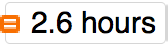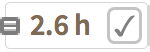Press the check mark to accept the interpretation: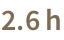You can use InputForm to see how the Wolfram Language internally represents this.
 In:=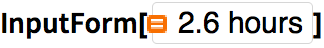Out=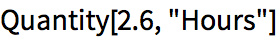You can always enter quantities directly like this, or you can use ctrl+=, either for the whole thing, or just for the units.
 In:=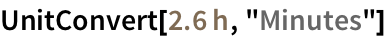Out=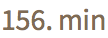In:=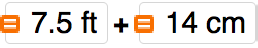Out=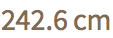Divide one length by another:
 In:=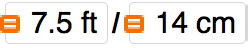Out=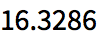Use dollars in a computation:
 In:=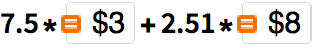Out=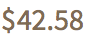In:=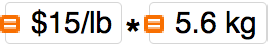Out=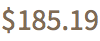In:=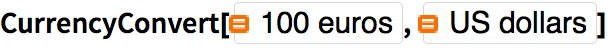Out=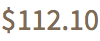In:=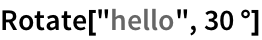Out=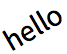If you leave off the Degree or °, the Wolfram Language will assume youre talking about radians, which go from 0 to 2π (about 6.28) around a circle, rather than degrees, which go from 0 to 360.
π/2 radians is equivalent to 90°:
 In:=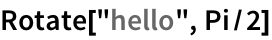Out=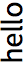Make a list of rotations in degrees from 0 to 360:
 In:=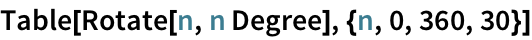Out=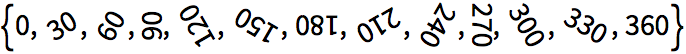Theres lots to do with angles. For example, AnglePath gives the path youd follow if you successively turned by a sequence of angles.
Start off horizontal, then turn three times by 80°:
 In:=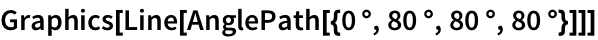Out=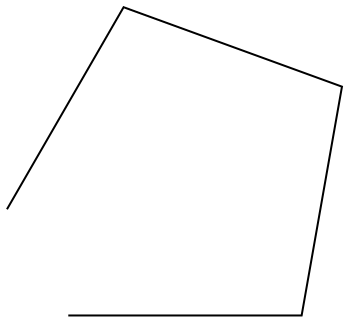Keep turning by 80° and youll eventually get back to where you started:
 In:=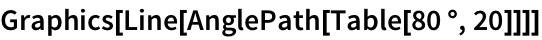Out=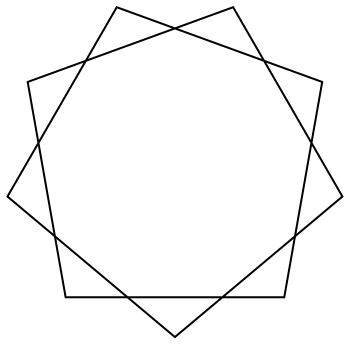In:=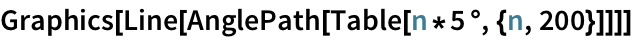Out=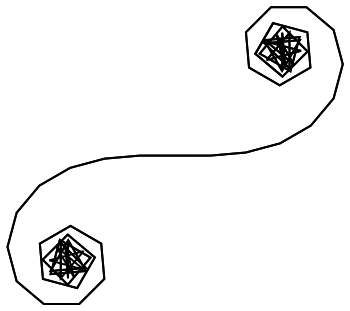17.1Convert 4.5 lbs (pounds) to kilograms. »
Expected output:
 Out[]=Answer & check your solution
17.2Convert 60.25 mph to kilometers per hour. »
Expected output:
 Out[]=Answer & check your solution
17.3Find the height of the Eiffel Tower in miles. »
Expected output:
 Out[]=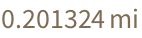Answer & check your solution
17.4Find the height of Mount Everest divided by the height of the Eiffel Tower. »
Expected output:
 Out[]=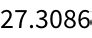Answer & check your solution
17.5Find the mass of the Earth divided by the mass of the Moon. »
Expected output:
 Out[]=Answer & check your solution
17.6Convert 2500 Japanese yen to US dollars. »
Sample expected output:
 Out[]=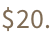Answer & check your solution
17.7Find the total of 35 ounces, 1/4 ton, 45 lbs and 9 stone in kilograms. »
Expected output:
 Out[]=Answer & check your solution
17.8Get a list of the distances to each planet using the "DistanceFromEarth" property, and convert all the results to light minutes. »
Expected output:
 Out[]=Answer & check your solution
17.9Rotate the string "hello" by 180°»
Expected output:
 Out[]=Answer & check your solution
17.10Make a table of a size-100 A rotated by 0° through 360° in steps of 30°»
Expected output:
 Out[]=Answer & check your solution
17.11Make a Manipulate to rotate an image of a cat between 0° and 180°»
Sample expected output:
 Out[]=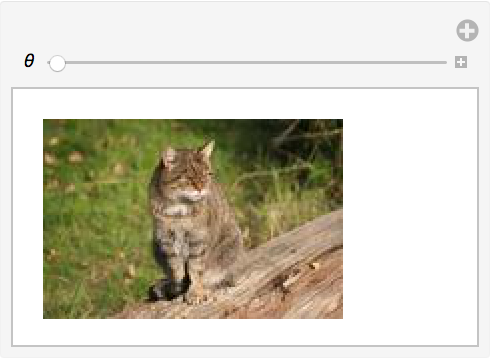Answer & check your solution
17.12Generate graphics for a path obtained by turning 0°, 1°, 2°, ... , 180°»
Expected output:
 Out[]=Answer & check your solution
17.13Make graphics of the path obtained by turning a constant angle 100 times, controlling the angle from 0° to 360° with a Manipulate»
Sample expected output:
 Out[]=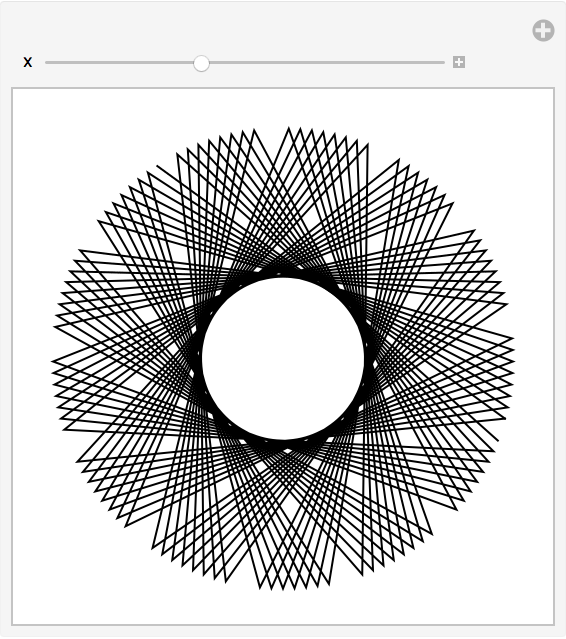Answer & check your solution
17.14Make graphics of the path obtained by successively turning by the digits of 2^10000 multiplied by 30°»
Sample expected output:
 Out[]=Answer & check your solution
+17.1Convert 4.3 light years to furlongs. »
Expected output:
 Out[]=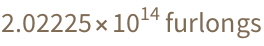Answer & check your solution
+17.2Convert 20,000 leagues to miles. »
Expected output:
 Out[]=Answer & check your solution
+17.3Make a Manipulate to rotate a size-200 W to any angle from 0° to 360°»
Sample expected output:
 Out[]=Answer & check your solution
+17.4Rotate an image of the Great Pyramid by 180°»
Sample expected output:
 Out[]=Answer & check your solution
+17.5Generate a list of letters of the alphabet, each randomly rotated. »
Sample expected output:
 Out[]=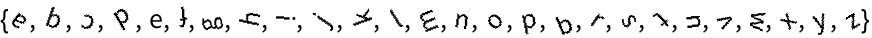Answer & check your solution
+17.6Generate graphics for a path obtained by turning 100 times through a random number of degrees between 0 and 360. »
Sample expected output:
 Out[]=Answer & check your solution
What unit abbreviations does the Wolfram Language understand?
Does the Wolfram Language pick units based on what country Im in?
Only to interpret input like 5 kg. If you type Quantity[5, "Kilograms"] you dont need the networkexcept to deal with units like currencies whose values are always changing.
What can I do if my unit conversion gives me an exact fraction but I want a decimal number?
Use the function N[...] to find a decimal approximation. Or add a decimal point to a number in your input. Well talk more about this in Section 23.
Why dont I get the same result for the currency conversion example?